Publicité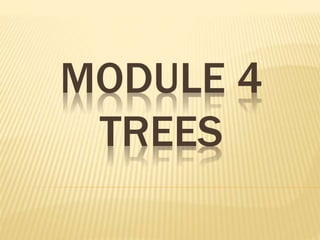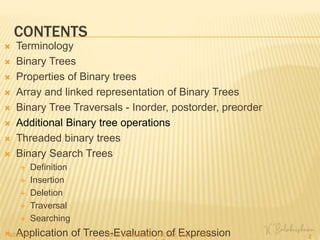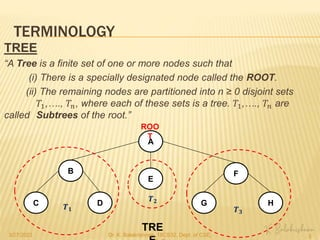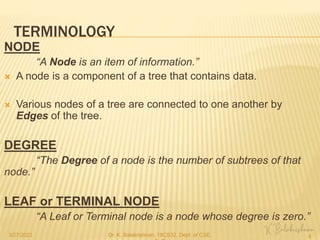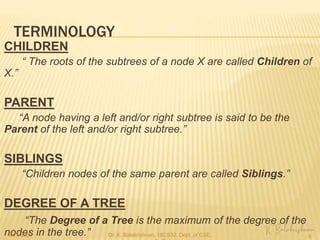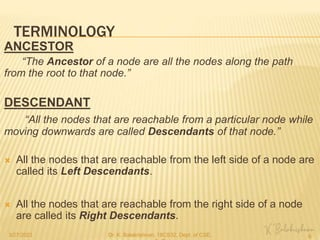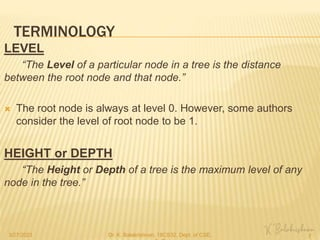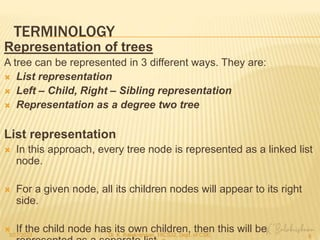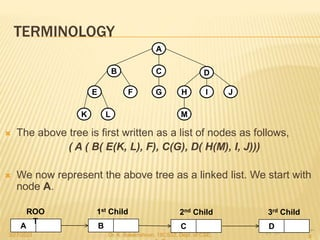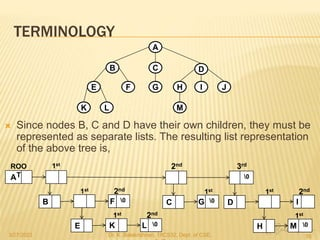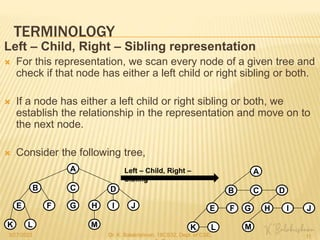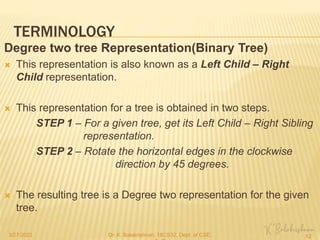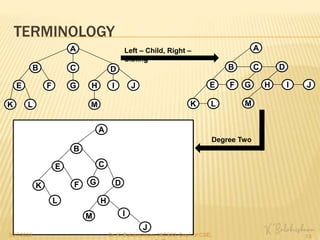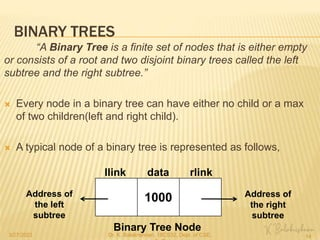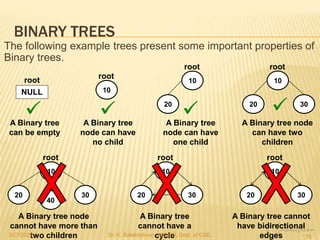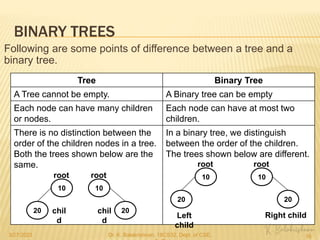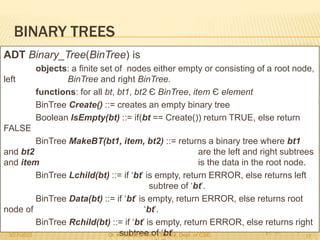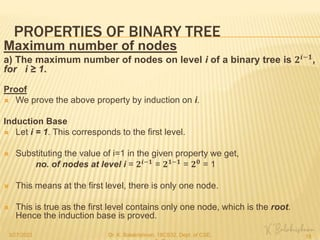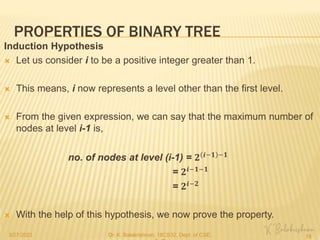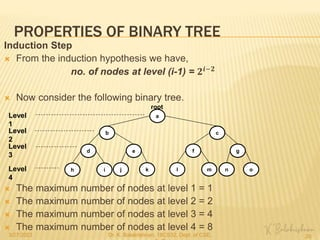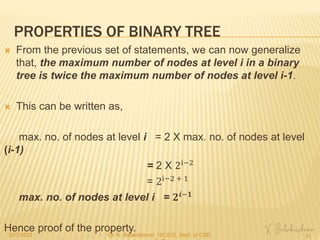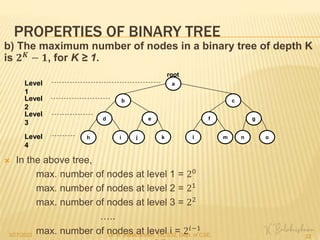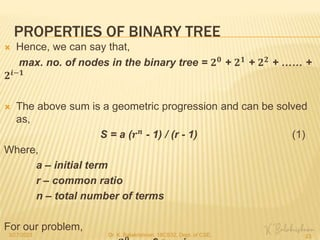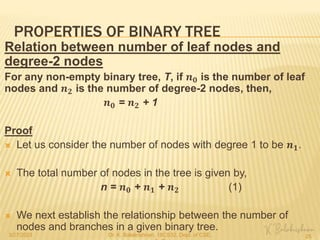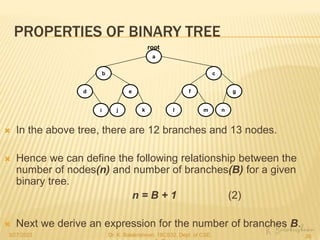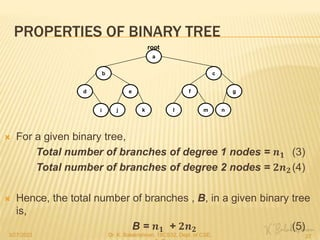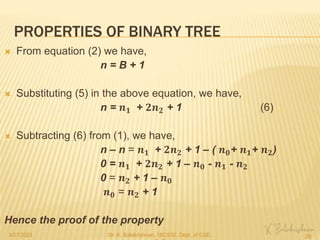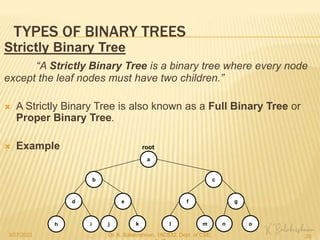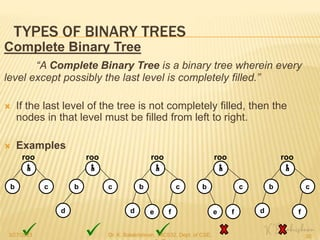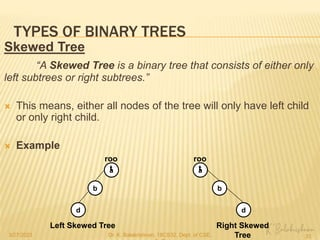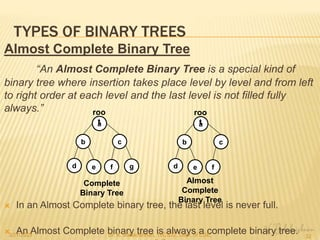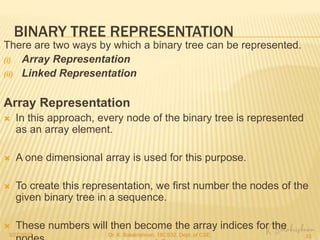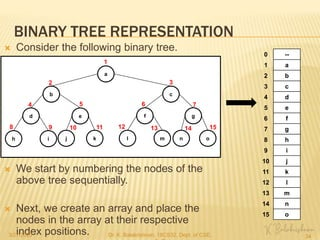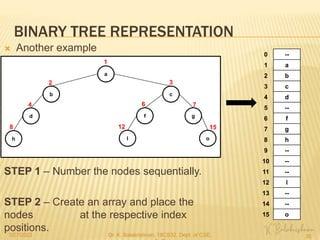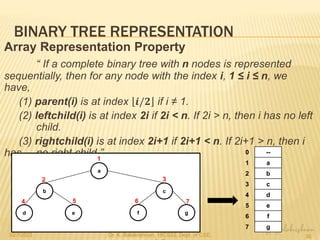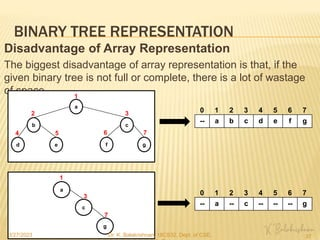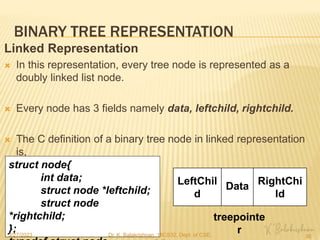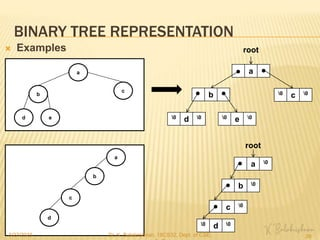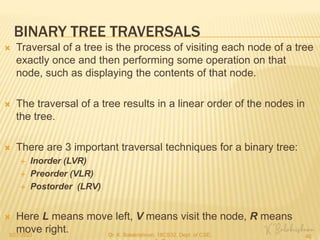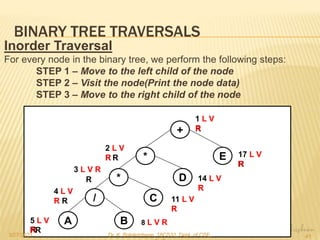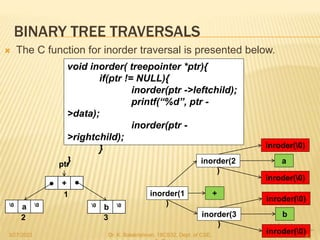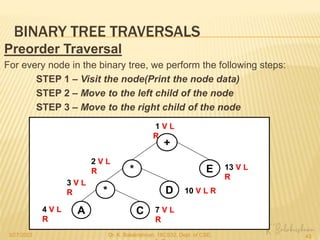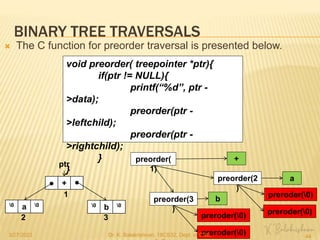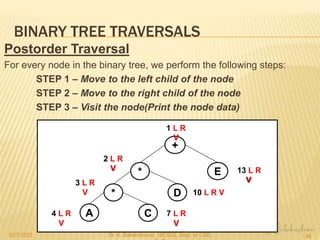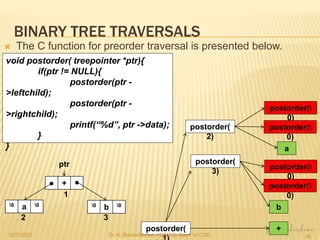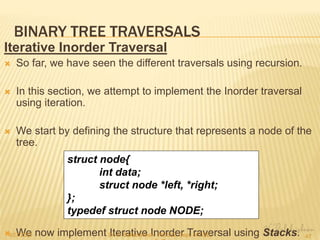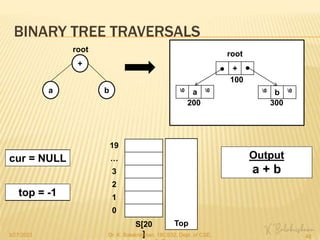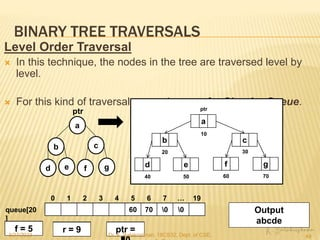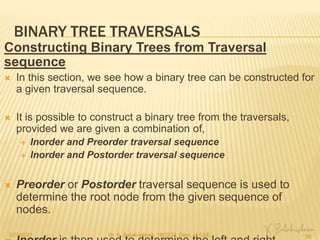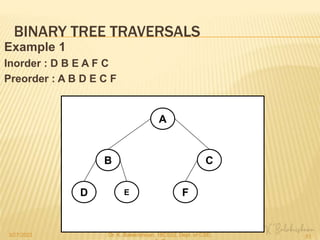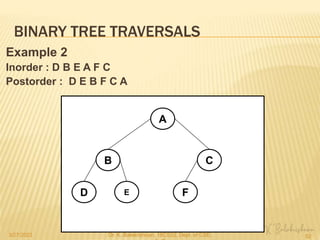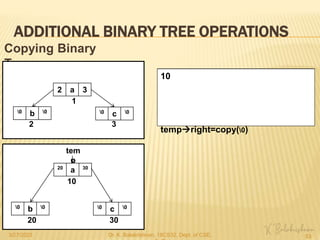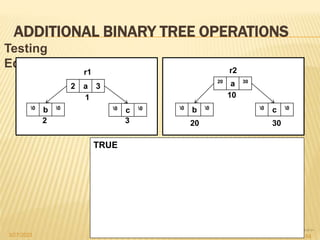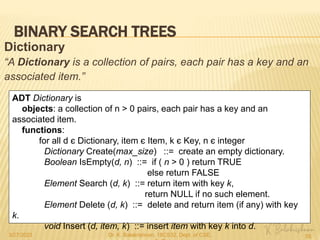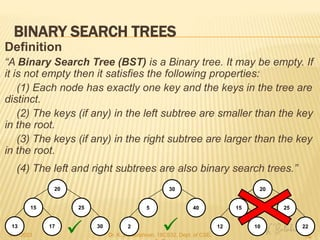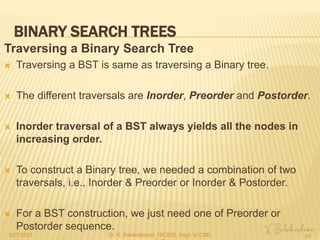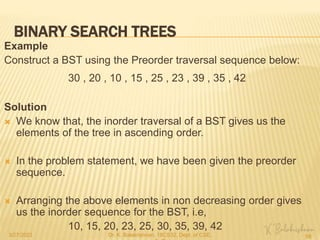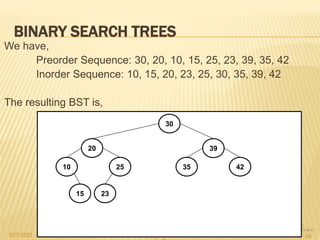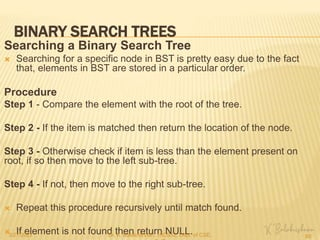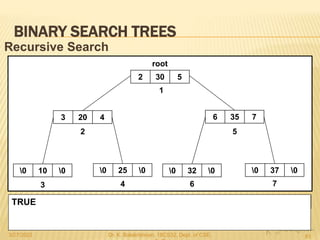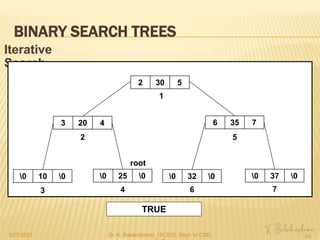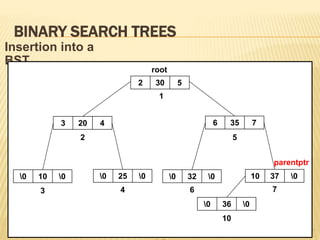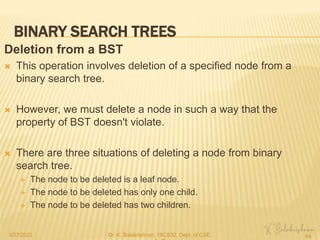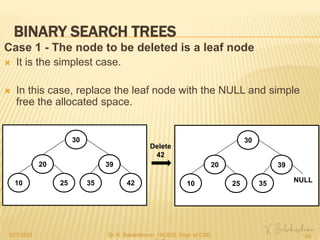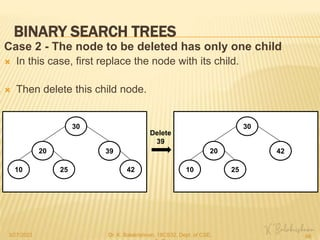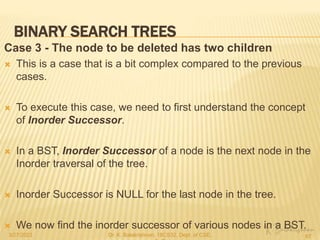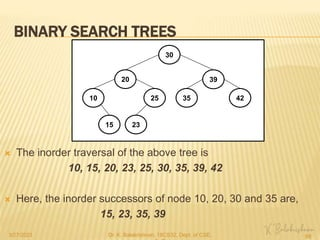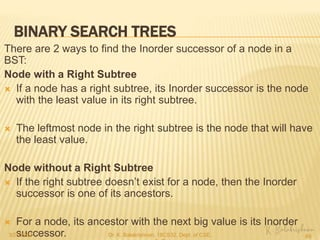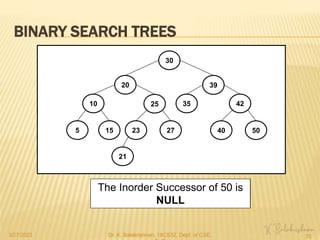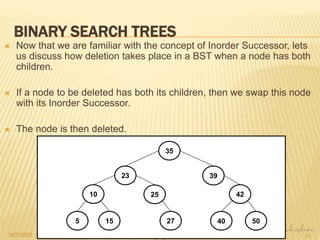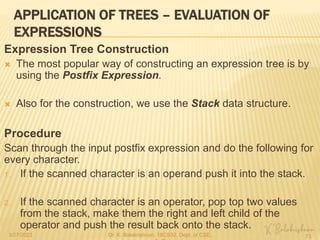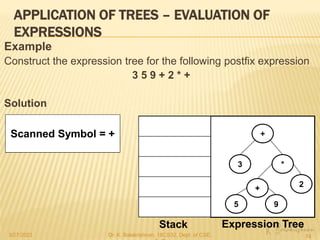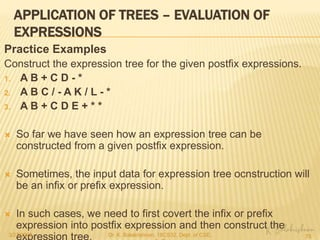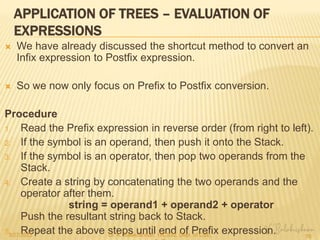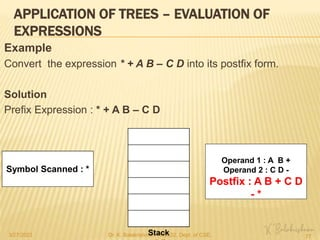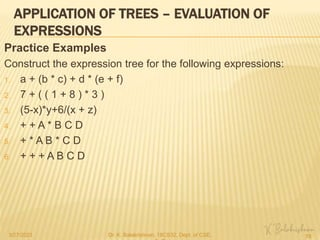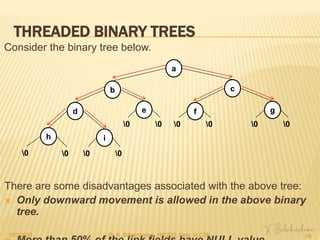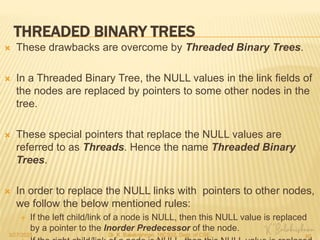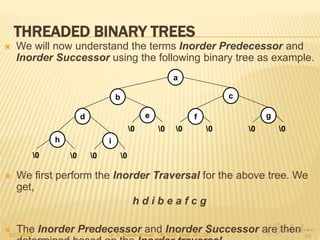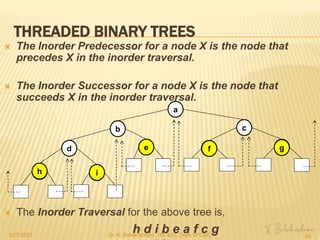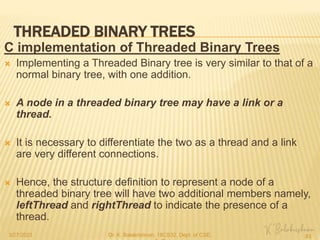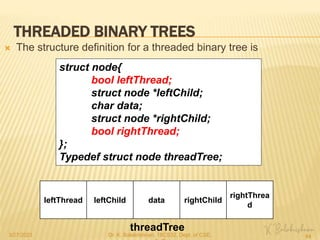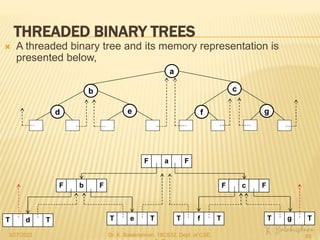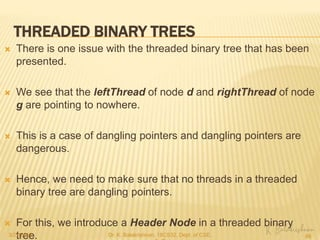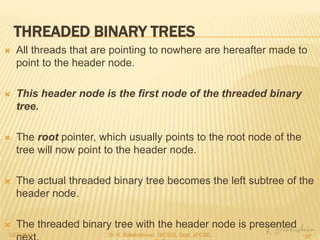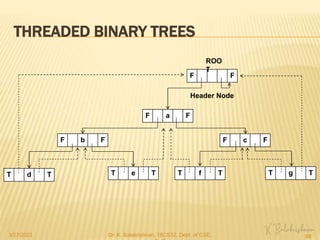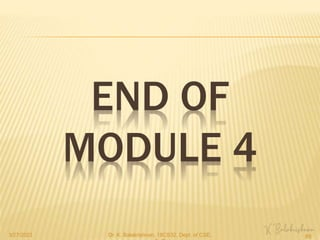1 sur 89
Publicité

### Module 4.pptx

1. MODULE 4 TREES
2. CONTENTS  Terminology  Binary Trees  Properties of Binary trees  Array and linked representation of Binary Trees  Binary Tree Traversals - Inorder, postorder, preorder  Additional Binary tree operations  Threaded binary trees  Binary Search Trees  Definition  Insertion  Deletion  Traversal  Searching  Application of Trees-Evaluation of Expression 3/27/2023 Dr. K. Balakrishnan, 18CS32, Dept. of CSE, 2
3. TERMINOLOGY TREE “A Tree is a finite set of one or more nodes such that (i) There is a specially designated node called the ROOT. (ii) The remaining nodes are partitioned into n ≥ 0 disjoint sets 𝑇1,…., 𝑇𝑛, where each of these sets is a tree. 𝑇1,…., 𝑇𝑛 are called Subtrees of the root.” A B C D E F G H TRE ROO T 𝑻𝟏 𝑻𝟐 𝑻𝟑 3/27/2023 Dr. K. Balakrishnan, 18CS32, Dept. of CSE, 3
4. TERMINOLOGY NODE “A Node is an item of information.”  A node is a component of a tree that contains data.  Various nodes of a tree are connected to one another by Edges of the tree. DEGREE “The Degree of a node is the number of subtrees of that node.” LEAF or TERMINAL NODE “A Leaf or Terminal node is a node whose degree is zero.” 3/27/2023 Dr. K. Balakrishnan, 18CS32, Dept. of CSE, 4
5. TERMINOLOGY CHILDREN “ The roots of the subtrees of a node X are called Children of X.” PARENT “A node having a left and/or right subtree is said to be the Parent of the left and/or right subtree.” SIBLINGS “Children nodes of the same parent are called Siblings.” DEGREE OF A TREE “The Degree of a Tree is the maximum of the degree of the nodes in the tree.” 3/27/2023 Dr. K. Balakrishnan, 18CS32, Dept. of CSE, 5
6. TERMINOLOGY ANCESTOR “The Ancestor of a node are all the nodes along the path from the root to that node.” DESCENDANT “All the nodes that are reachable from a particular node while moving downwards are called Descendants of that node.”  All the nodes that are reachable from the left side of a node are called its Left Descendants.  All the nodes that are reachable from the right side of a node are called its Right Descendants. 3/27/2023 Dr. K. Balakrishnan, 18CS32, Dept. of CSE, 6
7. TERMINOLOGY LEVEL “The Level of a particular node in a tree is the distance between the root node and that node.”  The root node is always at level 0. However, some authors consider the level of root node to be 1. HEIGHT or DEPTH “The Height or Depth of a tree is the maximum level of any node in the tree.” 3/27/2023 Dr. K. Balakrishnan, 18CS32, Dept. of CSE, 7
8. TERMINOLOGY Representation of trees A tree can be represented in 3 different ways. They are:  List representation  Left – Child, Right – Sibling representation  Representation as a degree two tree List representation  In this approach, every tree node is represented as a linked list node.  For a given node, all its children nodes will appear to its right side.  If the child node has its own children, then this will be 3/27/2023 Dr. K. Balakrishnan, 18CS32, Dept. of CSE, 8
9. TERMINOLOGY  The above tree is first written as a list of nodes as follows, ( A ( B( E(K, L), F), C(G), D( H(M), I, J)))  We now represent the above tree as a linked list. We start with node A. A B E F C D H J G I K L M A ROO T B 1st Child C 2nd Child D 3rd Child 3/27/2023 Dr. K. Balakrishnan, 18CS32, Dept. of CSE, 9
10. TERMINOLOGY  Since nodes B, C and D have their own children, they must be represented as separate lists. The resulting list representation of the above tree is, A B E F C D H J G I K L M A ROO T 1st 2nd 0 3rd B F 0 E K L 0 1st 2nd 1st 2nd C G 0 1st D I 1st 2nd H M 0 1st 3/27/2023 Dr. K. Balakrishnan, 18CS32, Dept. of CSE, 10
11. TERMINOLOGY Left – Child, Right – Sibling representation  For this representation, we scan every node of a given tree and check if that node has either a left child or right sibling or both.  If a node has either a left child or right sibling or both, we establish the relationship in the representation and move on to the next node.  Consider the following tree, A B E F C D H J G I K L M Left – Child, Right – Sibling A B E F C D H J G I K L M 3/27/2023 Dr. K. Balakrishnan, 18CS32, Dept. of CSE, 11
12. TERMINOLOGY Degree two tree Representation(Binary Tree)  This representation is also known as a Left Child – Right Child representation.  This representation for a tree is obtained in two steps. STEP 1 – For a given tree, get its Left Child – Right Sibling representation. STEP 2 – Rotate the horizontal edges in the clockwise direction by 45 degrees.  The resulting tree is a Degree two representation for the given tree. 3/27/2023 Dr. K. Balakrishnan, 18CS32, Dept. of CSE, 12
13. TERMINOLOGY A B E F C D H J G I K L M Left – Child, Right – Sibling A B E F C D H J G I K L M Degree Two A B E F C D H J G I K L M A B E F C D H J G I K L M A B E F C D H J G I K L M A B E F C D H J G I K L M A B E F C D H J G I K L M A B E F C D H J G I K L M 3/27/2023 Dr. K. Balakrishnan, 18CS32, Dept. of CSE, 13
14. BINARY TREES “A Binary Tree is a finite set of nodes that is either empty or consists of a root and two disjoint binary trees called the left subtree and the right subtree.”  Every node in a binary tree can have either no child or a max of two children(left and right child).  A typical node of a binary tree is represented as follows, 1000 llink data rlink Address of the left subtree Address of the right subtree Binary Tree Node 3/27/2023 Dr. K. Balakrishnan, 18CS32, Dept. of CSE, 14
15. BINARY TREES The following example trees present some important properties of Binary trees. NULL root A Binary tree can be empty 10 root A Binary tree node can have no child 10 root 20 A Binary tree node can have one child A Binary tree node can have two children 10 root 20 30 10 root 20 30 40 A Binary tree node cannot have more than two children 10 root 20 30 A Binary tree cannot have a cycle 10 root 20 30 A Binary tree cannot have bidirectional edges 3/27/2023 Dr. K. Balakrishnan, 18CS32, Dept. of CSE, 15
16. BINARY TREES Following are some points of difference between a tree and a binary tree. Tree Binary Tree A Tree cannot be empty. A Binary tree can be empty Each node can have many children or nodes. Each node can have at most two children. There is no distinction between the order of the children nodes in a tree. Both the trees shown below are the same. In a binary tree, we distinguish between the order of the children. The trees shown below are different. 10 root 20 10 root 20 10 root 20 10 root 20 Left child Right child chil d chil d 3/27/2023 Dr. K. Balakrishnan, 18CS32, Dept. of CSE, 16
17. BINARY TREES ADT Binary_Tree(BinTree) is objects: a finite set of nodes either empty or consisting of a root node, left BinTree and right BinTree. functions: for all bt, bt1, bt2 Є BinTree, item Є element BinTree Create() ::= creates an empty binary tree Boolean IsEmpty(bt) ::= if(bt == Create()) return TRUE, else return FALSE BinTree MakeBT(bt1, item, bt2) ::= returns a binary tree where bt1 and bt2 are the left and right subtrees and item is the data in the root node. BinTree Lchild(bt) ::= if ‘bt’ is empty, return ERROR, else returns left subtree of ‘bt’. BinTree Data(bt) ::= if ‘bt’ is empty, return ERROR, else returns root node of ‘bt’. BinTree Rchild(bt) ::= if ‘bt’ is empty, return ERROR, else returns right subtree of ‘bt’. 3/27/2023 Dr. K. Balakrishnan, 18CS32, Dept. of CSE, 17
18. PROPERTIES OF BINARY TREE Maximum number of nodes a) The maximum number of nodes on level i of a binary tree is 𝟐𝒊−𝟏 , for i ≥ 1. Proof  We prove the above property by induction on i. Induction Base  Let i = 1. This corresponds to the first level.  Substituting the value of i=1 in the given property we get, no. of nodes at level i = 𝟐𝒊−𝟏 = 𝟐𝟏−𝟏 = 𝟐𝟎 = 1  This means at the first level, there is only one node.  This is true as the first level contains only one node, which is the root. Hence the induction base is proved. 3/27/2023 Dr. K. Balakrishnan, 18CS32, Dept. of CSE, 18
19. PROPERTIES OF BINARY TREE Induction Hypothesis  Let us consider i to be a positive integer greater than 1.  This means, i now represents a level other than the first level.  From the given expression, we can say that the maximum number of nodes at level i-1 is, no. of nodes at level (i-1) = 𝟐 𝒊−𝟏 −𝟏 = 𝟐𝒊−𝟏−𝟏 = 𝟐𝒊−𝟐  With the help of this hypothesis, we now prove the property. 3/27/2023 Dr. K. Balakrishnan, 18CS32, Dept. of CSE, 19
20. PROPERTIES OF BINARY TREE Induction Step  From the induction hypothesis we have, no. of nodes at level (i-1) = 𝟐𝒊−𝟐  Now consider the following binary tree.  The maximum number of nodes at level 1 = 1  The maximum number of nodes at level 2 = 2  The maximum number of nodes at level 3 = 4  The maximum number of nodes at level 4 = 8 a root b c d e f g h i j k l m n o Level 1 Level 2 Level 3 Level 4 3/27/2023 Dr. K. Balakrishnan, 18CS32, Dept. of CSE, 20
21. PROPERTIES OF BINARY TREE  From the previous set of statements, we can now generalize that, the maximum number of nodes at level i in a binary tree is twice the maximum number of nodes at level i-1.  This can be written as, max. no. of nodes at level i = 2 X max. no. of nodes at level (i-1) = 2 X 2i−2 = 2i−2 + 1 max. no. of nodes at level i = 𝟐𝒊−𝟏 Hence proof of the property. 3/27/2023 Dr. K. Balakrishnan, 18CS32, Dept. of CSE, 21
22. PROPERTIES OF BINARY TREE b) The maximum number of nodes in a binary tree of depth K is 𝟐𝑲 − 𝟏, for K ≥ 1. a root b c d e f g h i j k l m n o Level 1 Level 2 Level 3 Level 4  In the above tree, max. number of nodes at level 1 = 20 max. number of nodes at level 2 = 21 max. number of nodes at level 3 = 22 ….. max. number of nodes at level i = 2𝑖−1 3/27/2023 Dr. K. Balakrishnan, 18CS32, Dept. of CSE, 22
23. PROPERTIES OF BINARY TREE  Hence, we can say that, max. no. of nodes in the binary tree = 𝟐𝟎 + 𝟐𝟏 + 𝟐𝟐 + …… + 𝟐𝒊−𝟏  The above sum is a geometric progression and can be solved as, S = a (𝒓𝒏 - 1) / (r - 1) (1) Where, a – initial term r – common ratio n – total number of terms For our problem, 3/27/2023 Dr. K. Balakrishnan, 18CS32, Dept. of CSE, 23
24. PROPERTIES OF BINARY TREE  Substituting the values in (1) , we get, S = 𝟐𝟎 (𝟐𝒊 - 1) / (2 - 1) = 𝟐𝒊 - 1  Since S is the sum that corresponds to the maximum number of nodes in the binary tree, we can write the above expression as, max. no. of nodes in the binary tree = 𝟐𝒊 - 1 (2)  The depth of a tree is denoted by K. Depth of a tree is the maximum level of the tree.  In our example, the maximum level is denoted by i. Hence, K = i  Making this substitution in (2) we have, max. no. of nodes in the binary tree of depth K = 𝟐𝑲 - 1 3/27/2023 Dr. K. Balakrishnan, 18CS32, Dept. of CSE, 24
25. PROPERTIES OF BINARY TREE Relation between number of leaf nodes and degree-2 nodes For any non-empty binary tree, T, if 𝒏𝟎 is the number of leaf nodes and 𝒏𝟐 is the number of degree-2 nodes, then, 𝒏𝟎 = 𝒏𝟐 + 1 Proof  Let us consider the number of nodes with degree 1 to be 𝒏𝟏.  The total number of nodes in the tree is given by, n = 𝒏𝟎 + 𝒏𝟏 + 𝒏𝟐 (1)  We next establish the relationship between the number of nodes and branches in a given binary tree. 3/27/2023 Dr. K. Balakrishnan, 18CS32, Dept. of CSE, 25
26. PROPERTIES OF BINARY TREE  In the above tree, there are 12 branches and 13 nodes.  Hence we can define the following relationship between the number of nodes(n) and number of branches(B) for a given binary tree. n = B + 1 (2)  Next we derive an expression for the number of branches B. a root b c d e f g i j k l m n 3/27/2023 Dr. K. Balakrishnan, 18CS32, Dept. of CSE, 26
27. PROPERTIES OF BINARY TREE  For a given binary tree, Total number of branches of degree 1 nodes = 𝒏𝟏 (3) Total number of branches of degree 2 nodes = 𝟐𝒏𝟐 (4)  Hence, the total number of branches , B, in a given binary tree is, B = 𝒏𝟏 + 𝟐𝒏𝟐 (5) a root b c d e f g i j k l m n 3/27/2023 Dr. K. Balakrishnan, 18CS32, Dept. of CSE, 27
28. PROPERTIES OF BINARY TREE  From equation (2) we have, n = B + 1  Substituting (5) in the above equation, we have, n = 𝒏𝟏 + 𝟐𝒏𝟐 + 1 (6)  Subtracting (6) from (1), we have, n – n = 𝒏𝟏 + 𝟐𝒏𝟐 + 1 – ( 𝒏𝟎+ 𝒏𝟏+ 𝒏𝟐) 0 = 𝒏𝟏 + 𝟐𝒏𝟐 + 1 – 𝒏𝟎 - 𝒏𝟏 - 𝒏𝟐 0 = 𝒏𝟐 + 1 – 𝒏𝟎 𝒏𝟎 = 𝒏𝟐 + 1 Hence the proof of the property 3/27/2023 Dr. K. Balakrishnan, 18CS32, Dept. of CSE, 28
29. TYPES OF BINARY TREES Strictly Binary Tree “A Strictly Binary Tree is a binary tree where every node except the leaf nodes must have two children.”  A Strictly Binary Tree is also known as a Full Binary Tree or Proper Binary Tree.  Example a root b c d e f g h i j k l m n o 3/27/2023 Dr. K. Balakrishnan, 18CS32, Dept. of CSE, 29
30. TYPES OF BINARY TREES Complete Binary Tree “A Complete Binary Tree is a binary tree wherein every level except possibly the last level is completely filled.”  If the last level of the tree is not completely filled, then the nodes in that level must be filled from left to right.  Examples a roo t b c a roo t b c d a roo t b c d e f a roo t b c e f a roo t b c d f 3/27/2023 Dr. K. Balakrishnan, 18CS32, Dept. of CSE, 30
31. TYPES OF BINARY TREES Skewed Tree “A Skewed Tree is a binary tree that consists of either only left subtrees or right subtrees.”  This means, either all nodes of the tree will only have left child or only right child.  Example a roo t b d a roo t b d Left Skewed Tree Right Skewed Tree 3/27/2023 Dr. K. Balakrishnan, 18CS32, Dept. of CSE, 31
32. TYPES OF BINARY TREES Almost Complete Binary Tree “An Almost Complete Binary Tree is a special kind of binary tree where insertion takes place level by level and from left to right order at each level and the last level is not filled fully always.” a roo t b c d e f g a roo t b c d e f Complete Binary Tree Almost Complete Binary Tree  In an Almost Complete binary tree, the last level is never full.  An Almost Complete binary tree is always a complete binary tree. 3/27/2023 Dr. K. Balakrishnan, 18CS32, Dept. of CSE, 32
33. BINARY TREE REPRESENTATION There are two ways by which a binary tree can be represented. (i) Array Representation (ii) Linked Representation Array Representation  In this approach, every node of the binary tree is represented as an array element.  A one dimensional array is used for this purpose.  To create this representation, we first number the nodes of the given binary tree in a sequence.  These numbers will then become the array indices for the 3/27/2023 Dr. K. Balakrishnan, 18CS32, Dept. of CSE, 33
34. BINARY TREE REPRESENTATION  Consider the following binary tree. a 1 b c d e f g h i j k l m n o  We start by numbering the nodes of the above tree sequentially.  Next, we create an array and place the nodes in the array at their respective index positions. 2 3 4 5 6 7 15 14 13 12 11 10 9 8 -- a b c d e f g h i j k l m n o 0 1 2 3 4 5 6 7 8 9 10 11 12 13 14 15 3/27/2023 Dr. K. Balakrishnan, 18CS32, Dept. of CSE, 34
35. BINARY TREE REPRESENTATION  Another example a 1 b c d f g h l o 2 3 4 6 7 15 12 8 STEP 1 – Number the nodes sequentially. STEP 2 – Create an array and place the nodes at the respective index positions. -- a b c d -- f g h -- -- -- l -- -- o 0 1 2 3 4 5 6 7 8 9 10 11 12 13 14 15 3/27/2023 Dr. K. Balakrishnan, 18CS32, Dept. of CSE, 35
36. BINARY TREE REPRESENTATION Array Representation Property “ If a complete binary tree with n nodes is represented sequentially, then for any node with the index i, 1 ≤ i ≤ n, we have, (1) parent(i) is at index 𝒊/𝟐 if i ≠ 1. (2) leftchild(i) is at index 2i if 2i < n. If 2i > n, then i has no left child. (3) rightchild(i) is at index 2i+1 if 2i+1 < n. If 2i+1 > n, then i has no right child.” a 1 b c d e f g 2 3 4 5 6 7 -- a b c d e f g 0 1 2 3 4 5 6 7 3/27/2023 Dr. K. Balakrishnan, 18CS32, Dept. of CSE, 36
37. BINARY TREE REPRESENTATION Disadvantage of Array Representation The biggest disadvantage of array representation is that, if the given binary tree is not full or complete, there is a lot of wastage of space. a 1 b c d e f g 2 3 4 5 6 7 a 1 c g 3 7 -- a b c d e f g 0 1 2 3 4 5 6 7 -- a -- c -- -- -- g 0 1 2 3 4 5 6 7 3/27/2023 Dr. K. Balakrishnan, 18CS32, Dept. of CSE, 37
38. BINARY TREE REPRESENTATION Linked Representation  In this representation, every tree node is represented as a doubly linked list node.  Every node has 3 fields namely data, leftchild, rightchild.  The C definition of a binary tree node in linked representation is, struct node{ int data; struct node *leftchild; struct node *rightchild; }; LeftChil d Data RightChi ld treepointe r 3/27/2023 Dr. K. Balakrishnan, 18CS32, Dept. of CSE, 38
39. BINARY TREE REPRESENTATION  Examples a b c d e a 0 c 0 b 0 d 0 0 e 0 root a b c d a 0 root b 0 c 0 0 d 0 3/27/2023 Dr. K. Balakrishnan, 18CS32, Dept. of CSE, 39
40. BINARY TREE TRAVERSALS  Traversal of a tree is the process of visiting each node of a tree exactly once and then performing some operation on that node, such as displaying the contents of that node.  The traversal of a tree results in a linear order of the nodes in the tree.  There are 3 important traversal techniques for a binary tree:  Inorder (LVR)  Preorder (VLR)  Postorder (LRV)  Here L means move left, V means visit the node, R means move right. 3/27/2023 Dr. K. Balakrishnan, 18CS32, Dept. of CSE, 40
41. D + E * * C / A B BINARY TREE TRAVERSALS Inorder Traversal For every node in the binary tree, we perform the following steps: STEP 1 – Move to the left child of the node STEP 2 – Visit the node(Print the node data) STEP 3 – Move to the right child of the node L V R L V R L V R L V R L V R L V R L V R L V R L V R 1 L V R 2 L V R 3 L V R 4 L V R 5 L V R 5 L V R 5 L V R 4 L V R 4 L V R 8 L V R 8 L V R 8 L V R 3 L V R 3 L V R 11 L V R 11 L V R 11 L V R 2 L V R 2 L V R 14 L V R 14 L V R 14 L V R 1 L V R 1 L V R 17 L V R 17 L V R 17 L V R 3/27/2023 Dr. K. Balakrishnan, 18CS32, Dept. of CSE, 41
42. BINARY TREE TRAVERSALS  The C function for inorder traversal is presented below. void inorder( treepointer *ptr){ if(ptr != NULL){ inorder(ptr ->leftchild); printf(“%d”, ptr - >data); inorder(ptr - >rightchild); } } + 0 b 0 0 a 0 ptr 1 2 3 inorder(1 ) inorder(2 ) inroder(0) a inroder(0) inorder(3 ) inroder(0) b inroder(0) + 3/27/2023 Dr. K. Balakrishnan, 18CS32, Dept. of CSE, 42
43. BINARY TREE TRAVERSALS Preorder Traversal For every node in the binary tree, we perform the following steps: STEP 1 – Visit the node(Print the node data) STEP 2 – Move to the left child of the node STEP 3 – Move to the right child of the node D + E * * C A V L R V L R V L R V L R V L R V L R V L R 1 V L R 1 V L R 2 V L R 2 V L R 3 V L R 3 V L R 4 V L R 4 V L R 4 V L R 3 V L R 7 V L R 7 V L R 7 V L R 2 V L R 10 V L R 10 V L R 10 V L R 1 V L R 13 V L R 13 V L R 13 V L R 3/27/2023 Dr. K. Balakrishnan, 18CS32, Dept. of CSE, 43
44. BINARY TREE TRAVERSALS  The C function for preorder traversal is presented below. void preorder( treepointer *ptr){ if(ptr != NULL){ printf(“%d”, ptr - >data); preorder(ptr - >leftchild); preorder(ptr - >rightchild); } } + 0 b 0 0 a 0 ptr 1 2 3 preorder( 1) + preorder(2 ) a preroder(0) preroder(0) preorder(3 ) b preroder(0) preroder(0) 3/27/2023 Dr. K. Balakrishnan, 18CS32, Dept. of CSE, 44
45. BINARY TREE TRAVERSALS Postorder Traversal For every node in the binary tree, we perform the following steps: STEP 1 – Move to the left child of the node STEP 2 – Move to the right child of the node STEP 3 – Visit the node(Print the node data) D + E * * C A L R V L R V L R V L R V L R V L R V L R V 1 L R V 2 L R V 3 L R V 4 L R V 4 L R V 4 L R V 3 L R V 7 L R V 7 L R V 7 L R V 3 L R V 2 L R V 10 L R V 10 L R V 10 L R V 2 L R V 1 L R V 13 L R V 13 L R V 13 L R V 1 L R V 3/27/2023 Dr. K. Balakrishnan, 18CS32, Dept. of CSE, 45
46. BINARY TREE TRAVERSALS  The C function for preorder traversal is presented below. void postorder( treepointer *ptr){ if(ptr != NULL){ postorder(ptr - >leftchild); postorder(ptr - >rightchild); printf(“%d”, ptr ->data); } } + 0 b 0 0 a 0 ptr 1 2 3 postorder( postorder( 2) postorder( 0) postorder( 0) a postorder( 3) postorder( 0) postorder( 0) b + 3/27/2023 Dr. K. Balakrishnan, 18CS32, Dept. of CSE, 46
47. BINARY TREE TRAVERSALS Iterative Inorder Traversal  So far, we have seen the different traversals using recursion.  In this section, we attempt to implement the Inorder traversal using iteration.  We start by defining the structure that represents a node of the tree. struct node{ int data; struct node *left, *right; }; typedef struct node NODE;  We now implement Iterative Inorder Traversal using Stacks. 3/27/2023 Dr. K. Balakrishnan, 18CS32, Dept. of CSE, 47
48. BINARY TREE TRAVERSALS + 0 b 0 0 a 0 root 100 200 300 + a b root S[20 ] 19 … 3 2 1 0 cur = top = -1 Top cur = 100 top = 0 Top 100 cur = 200 cur + 0 b 0 0 a 0 root 100 200 300 cur top = 1 Top 200 100 cur = NULL cur cur = 200 Top top = 0 100 Output a cur = NULL cur cur cur = 100 cur top = -1 Top Output a + cur = 300 cur cur top = 0 Top 300 cur = NULL cur cur = 300 cur top = -1 Top Output a + b cur = NULL cur 3/27/2023 Dr. K. Balakrishnan, 18CS32, Dept. of CSE, 48
49. BINARY TREE TRAVERSALS Level Order Traversal  In this technique, the nodes in the tree are traversed level by level.  For this kind of traversal, we make use of a Circular Queue. a b c ptr d e f g a c b ptr 20 30 d 40 e 50 f 60 g 70 10 0 1 2 3 4 5 6 7 … 19 queue[20 ] f = 0 r = 0 ptr = Output a 10 r = 1 f = 1 20 r = 2 20 30 r = 3 f = 2 30 Output ab r = 4 30 40 r = 5 30 40 50 ptr = f = 3 40 50 ptr = Output abc r = 6 40 50 60 r = 7 40 50 60 70 f = 4 ptr = 50 60 70 Output abcd r = 8 50 60 70 0 r = 9 50 60 70 0 0 f = 5 ptr = 60 70 0 0 Output abcde 3/27/2023 Dr. K. Balakrishnan, 18CS32, Dept. of CSE, 49
50. BINARY TREE TRAVERSALS Constructing Binary Trees from Traversal sequence  In this section, we see how a binary tree can be constructed for a given traversal sequence.  It is possible to construct a binary tree from the traversals, provided we are given a combination of,  Inorder and Preorder traversal sequence  Inorder and Postorder traversal sequence  Preorder or Postorder traversal sequence is used to determine the root node from the given sequence of nodes. 3/27/2023 Dr. K. Balakrishnan, 18CS32, Dept. of CSE, 50
51. BINARY TREE TRAVERSALS Example 1 Inorder : D B E A F C Preorder : A B D E C F A DBE FC A FC B D E A B D E C F 3/27/2023 Dr. K. Balakrishnan, 18CS32, Dept. of CSE, 51
52. BINARY TREE TRAVERSALS Example 2 Inorder : D B E A F C Postorder : D E B F C A A DBE FC A DBE C F A B D E C F 3/27/2023 Dr. K. Balakrishnan, 18CS32, Dept. of CSE, 52
53. a temp 10 ADDITIONAL BINARY TREE OPERATIONS Copying Binary Trees 3/27/2023 Dr. K. Balakrishnan, 18CS32, Dept. of CSE, 53 2 a 3 0 c 0 0 b 0 original 1 2 3 copy(1) copy(1) templeft = copy(2) 2 a 3 0 c 0 0 b 0 original 1 2 3 b temp 20 a 10 copy(1) templeft = copy(2) templeft = copy(0) 2 a 3 0 c 0 0 b 0 1 2 3 copy(1) templeft = copy(2) templeft = 0 0 b temp 20 a 10 copy(1) templeft = copy(2) templeft = 0 tempright = copy(0) original 2 a 3 0 c 0 0 b 0 1 2 3 copy(1) templeft = copy(2) templeft = 0 tempright = 0 0 b 0 temp 20 a 10 copy(1) templeft = 20 0 b 0 temp 20 20 a 10 original copy(1) templeft = 20 tempright = copy(3) 2 a 3 0 c 0 0 b 0 1 2 3 original 0 b 0 tem p 20 20 a c 30 10 copy(1) templeft = 20 tempright = copy(3) templeft = copy(0) 2 a 3 0 c 0 0 b 0 1 2 3 copy(1) templeft = 20 tempright = copy(3) templeft = 0 0 c copy(1) templeft = 20 tempright = copy(3) templeft = 0 tempright=copy(0) original 2 a 3 0 c 0 0 b 0 1 2 3 copy(1) templeft = 20 tempright = copy(3) templeft = 0 tempright= 0 0 c 0 copy(1) templeft = 20 tempright = 30 0 b 0 tem p 20 20 a 30 30 10 0 c 0 10
54. ADDITIONAL BINARY TREE OPERATIONS Testing Equality 3/27/2023 Dr. K. Balakrishnan, 18CS32, Dept. of CSE, SaIT 54 2 a 3 0 c 0 0 b 0 1 2 3 0 b 0 r2 20 20 a 30 30 10 0 c 0 r1 equal(1, 10) equal(1, 10) return ( equal(1, 10) return ( TRUE && equal(1, 10) return ( TRUE && equal(2, 20) 2 a 3 0 c 0 0 b 0 1 2 3 0 b 0 r2 20 20 a 30 30 10 0 c 0 r1 equal(1, 10) return ( TRUE && equal(2, 20) return( equal(1, 10) return ( TRUE && equal(2, 20) return( TRUE && equal(1, 10) return ( TRUE && equal(2, 20) return( TRUE && equal(0, 0) 2 a 3 0 c 0 0 b 0 1 2 3 0 b 0 r2 20 20 a 30 30 10 0 c 0 r1 equal(1, 10) return ( TRUE && equal(2, 20) return( TRUE && TRUE && equal(1, 10) return ( TRUE && equal(2, 20) return( TRUE && TRUE && equal(0, 0); 2 a 3 0 c 0 0 b 0 1 2 3 0 b 0 r2 20 20 a 30 30 10 0 c 0 r1 equal(1, 10) return ( TRUE && equal(2, 20) return( TRUE && TRUE && TRUE ); equal(1, 10) return ( TRUE && TRUE && 2 a 3 0 c 0 0 b 0 1 2 3 0 b 0 r2 20 20 a 30 30 10 0 c 0 r1 equal(1, 10) return ( TRUE && TRUE && equal(3, 30) 2 a 3 0 c 0 0 b 0 1 2 3 0 b 0 r2 20 20 a 30 30 10 0 c 0 r1 equal(1, 10) return ( TRUE && TRUE && equal(3, 30) return ( TRUE && equal(1, 10) return ( TRUE && TRUE && equal(3, 30) return ( TRUE && equal(0, 0) 2 a 3 0 c 0 0 b 0 1 2 3 0 b 0 r2 20 20 a 30 30 10 0 c 0 r1 equal(1, 10) return ( TRUE && TRUE && equal(3, 30) return ( TRUE && TRUE && equal(1, 10) return ( TRUE && TRUE && equal(3, 30) return ( TRUE && TRUE && equal(0, 0) 2 a 3 0 c 0 0 b 0 1 2 3 0 b 0 r2 20 20 a 30 30 10 0 c 0 r1 equal(1, 10) return ( TRUE && TRUE && equal(3, 30) return ( TRUE && TRUE && TRUE ); equal(1, 10) return ( TRUE && TRUE && TRUE); 2 a 3 0 c 0 0 b 0 1 2 3 0 b 0 r2 20 20 a 30 30 10 0 c 0 r1 TRUE
55. BINARY SEARCH TREES Dictionary “A Dictionary is a collection of pairs, each pair has a key and an associated item.” 3/27/2023 Dr. K. Balakrishnan, 18CS32, Dept. of CSE, 55 ADT Dictionary is objects: a collection of n > 0 pairs, each pair has a key and an associated item. functions: for all d є Dictionary, item є Item, k є Key, n є integer Dictionary Create(max_size) ::= create an empty dictionary. Boolean IsEmpty(d, n) ::= if ( n > 0 ) return TRUE else return FALSE Element Search (d, k) ::= return item with key k, return NULL if no such element. Element Delete (d, k) ::= delete and return item (if any) with key k. void Insert (d, item, k) ::= insert item with key k into d.
56. BINARY SEARCH TREES Definition “A Binary Search Tree (BST) is a Binary tree. It may be empty. If it is not empty then it satisfies the following properties: (1) Each node has exactly one key and the keys in the tree are distinct. (2) The keys (if any) in the left subtree are smaller than the key in the root. (3) The keys (if any) in the right subtree are larger than the key in the root. (4) The left and right subtrees are also binary search trees.” 3/27/2023 Dr. K. Balakrishnan, 18CS32, Dept. of CSE, 56 20 15 13 17 25 30 20 15 12 10 25 22 30 5 2 40
57. BINARY SEARCH TREES Traversing a Binary Search Tree  Traversing a BST is same as traversing a Binary tree.  The different traversals are Inorder, Preorder and Postorder.  Inorder traversal of a BST always yields all the nodes in increasing order.  To construct a Binary tree, we needed a combination of two traversals, i.e., Inorder & Preorder or Inorder & Postorder.  For a BST construction, we just need one of Preorder or Postorder sequence. 3/27/2023 Dr. K. Balakrishnan, 18CS32, Dept. of CSE, 57
58. BINARY SEARCH TREES Example Construct a BST using the Preorder traversal sequence below: 30 , 20 , 10 , 15 , 25 , 23 , 39 , 35 , 42 Solution  We know that, the inorder traversal of a BST gives us the elements of the tree in ascending order.  In the problem statement, we have been given the preorder sequence.  Arranging the above elements in non decreasing order gives us the inorder sequence for the BST, i.e, 10, 15, 20, 23, 25, 30, 35, 39, 42 3/27/2023 Dr. K. Balakrishnan, 18CS32, Dept. of CSE, 58
59. BINARY SEARCH TREES We have, Preorder Sequence: 30, 20, 10, 15, 25, 23, 39, 35, 42 Inorder Sequence: 10, 15, 20, 23, 25, 30, 35, 39, 42 The resulting BST is, 3/27/2023 Dr. K. Balakrishnan, 18CS32, Dept. of CSE, 59 30 10, 15, 20, 23, 25 35, 39, 42 30 10, 15 35, 39, 42 20 23, 25 30 10 35, 39, 42 20 23, 25 15 30 10 35, 39, 42 20 25 15 23 30 10 39 20 25 15 23 35 42
60. BINARY SEARCH TREES Searching a Binary Search Tree  Searching for a specific node in BST is pretty easy due to the fact that, elements in BST are stored in a particular order. Procedure Step 1 - Compare the element with the root of the tree. Step 2 - If the item is matched then return the location of the node. Step 3 - Otherwise check if item is less than the element present on root, if so then move to the left sub-tree. Step 4 - If not, then move to the right sub-tree.  Repeat this procedure recursively until match found.  If element is not found then return NULL. 3/27/2023 Dr. K. Balakrishnan, 18CS32, Dept. of CSE, 60
61. BINARY SEARCH TREES Recursive Search 3/27/2023 Dr. K. Balakrishnan, 18CS32, Dept. of CSE, 61 2 30 5 3 20 4 6 35 7 0 10 0 0 25 0 0 32 0 0 37 0 1 2 5 3 4 6 7 root Key = 25 Search(1, 25) Search(1, 25) Search(2 , 25) 2 30 5 3 20 4 6 35 7 0 10 0 0 25 0 0 32 0 0 37 0 1 2 5 3 4 6 7 root Search(1, 25) Search(2 , 25) Search(4, 25) 2 30 5 3 20 4 6 35 7 0 10 0 0 25 0 0 32 0 0 37 0 1 2 5 3 4 6 7 root Search(1, 25) Search(2 , 25) TRUE 2 30 5 3 20 4 6 35 7 0 10 0 0 25 0 0 32 0 0 37 0 1 2 5 3 4 6 7 root Search(1, 25) TRUE 2 30 5 3 20 4 6 35 7 0 10 0 0 25 0 0 32 0 0 37 0 1 2 5 3 4 6 7 root TRUE
62. BINARY SEARCH TREES Iterative Search 3/27/2023 Dr. K. Balakrishnan, 18CS32, Dept. of CSE, 62 2 30 5 3 20 4 6 35 7 0 10 0 0 25 0 0 32 0 0 37 0 1 2 5 3 4 6 7 root Key = 25 2 30 5 3 20 4 6 35 7 0 10 0 0 25 0 0 32 0 0 37 0 1 2 5 3 4 6 7 root 2 30 5 3 20 4 6 35 7 0 10 0 0 25 0 0 32 0 0 37 0 1 2 5 3 4 6 7 root TRUE
63. BINARY SEARCH TREES Insertion into a BST 3/27/2023 Dr. K. Balakrishnan, 18CS32, Dept. of CSE, 63 2 30 5 3 20 4 6 35 7 0 10 0 0 25 0 0 32 0 0 37 0 1 2 5 3 4 6 7 root 0 36 0 10 ptr nodeptr parentptr 2 30 5 3 20 4 6 35 7 0 10 0 0 25 0 0 32 0 0 37 0 1 2 5 3 4 6 7 root 0 36 0 10 ptr nodeptr parentptr 2 30 5 3 20 4 6 35 7 0 10 0 0 25 0 0 32 0 0 37 0 1 2 5 3 4 6 7 root 0 36 0 10 ptr nodeptr parentptr 2 30 5 3 20 4 6 35 7 0 10 0 0 25 0 0 32 0 0 37 0 1 2 5 3 4 6 7 root 0 36 0 10 ptr nodeptr parentptr 2 30 5 3 20 4 6 35 7 0 10 0 0 25 0 0 32 0 0 37 0 1 2 5 3 4 6 7 root 0 36 0 10 ptr nodeptr parentptr 2 30 5 3 20 4 6 35 7 0 10 0 0 25 0 0 32 0 0 37 0 1 2 5 3 4 6 7 root 0 36 0 10 ptr parentptr 2 30 5 3 20 4 6 35 7 0 10 0 0 25 0 0 32 0 10 37 0 1 2 5 3 4 6 7 root 0 36 0 10 parentptr
64. BINARY SEARCH TREES Deletion from a BST  This operation involves deletion of a specified node from a binary search tree.  However, we must delete a node in such a way that the property of BST doesn't violate.  There are three situations of deleting a node from binary search tree.  The node to be deleted is a leaf node.  The node to be deleted has only one child.  The node to be deleted has two children. 3/27/2023 Dr. K. Balakrishnan, 18CS32, Dept. of CSE, 64
65. BINARY SEARCH TREES Case 1 - The node to be deleted is a leaf node  It is the simplest case.  In this case, replace the leaf node with the NULL and simple free the allocated space. 3/27/2023 Dr. K. Balakrishnan, 18CS32, Dept. of CSE, 65 30 10 39 20 25 35 42 Delete 42 30 10 39 20 25 35 42 30 10 39 20 25 35 NULL
66. BINARY SEARCH TREES Case 2 - The node to be deleted has only one child  In this case, first replace the node with its child.  Then delete this child node. 3/27/2023 Dr. K. Balakrishnan, 18CS32, Dept. of CSE, 66 30 10 39 20 25 42 Delete 39 30 10 42 20 25 39 30 10 42 20 25
67. BINARY SEARCH TREES Case 3 - The node to be deleted has two children  This is a case that is a bit complex compared to the previous cases.  To execute this case, we need to first understand the concept of Inorder Successor.  In a BST, Inorder Successor of a node is the next node in the Inorder traversal of the tree.  Inorder Successor is NULL for the last node in the tree.  We now find the inorder successor of various nodes in a BST. 3/27/2023 Dr. K. Balakrishnan, 18CS32, Dept. of CSE, 67
68. BINARY SEARCH TREES  The inorder traversal of the above tree is 10, 15, 20, 23, 25, 30, 35, 39, 42  Here, the inorder successors of node 10, 20, 30 and 35 are, 15, 23, 35, 39 3/27/2023 Dr. K. Balakrishnan, 18CS32, Dept. of CSE, 68 30 10 39 20 25 15 23 35 42
69. BINARY SEARCH TREES There are 2 ways to find the Inorder successor of a node in a BST: Node with a Right Subtree  If a node has a right subtree, its Inorder successor is the node with the least value in its right subtree.  The leftmost node in the right subtree is the node that will have the least value. Node without a Right Subtree  If the right subtree doesn’t exist for a node, then the Inorder successor is one of its ancestors.  For a node, its ancestor with the next big value is its Inorder successor. 3/27/2023 Dr. K. Balakrishnan, 18CS32, Dept. of CSE, 69
70. BINARY SEARCH TREES 3/27/2023 Dr. K. Balakrishnan, 18CS32, Dept. of CSE, 70 30 10 39 20 25 15 23 35 42 5 21 27 40 50 The Inorder Successor of 20 is 30 10 39 20 25 15 23 35 42 5 21 27 40 50 Right Subtree 30 10 39 20 25 15 23 35 42 5 21 27 40 50 Leftmost Node in Right Subtree The Inorder Successor of 20 is 21 The Inorder Successor of 5 is 30 10 39 20 25 15 23 35 42 5 21 27 40 50 Ancestor s 30 10 39 20 15 23 35 42 5 21 27 40 50 Ancestor with the next big value 25 The Inorder Successor of 5 is 10 30 10 39 20 15 23 35 42 5 21 27 40 50 25 The Inorder Successor of 39 is The Inorder Successor of 39 is 40 The Inorder Successor of 15 is The Inorder Successor of 15 is 20 The Inorder Successor of 50 is The Inorder Successor of 50 is NULL
71. BINARY SEARCH TREES  Now that we are familiar with the concept of Inorder Successor, lets us discuss how deletion takes place in a BST when a node has both children.  If a node to be deleted has both its children, then we swap this node with its Inorder Successor.  The node is then deleted. 3/27/2023 Dr. K. Balakrishnan, 18CS32, Dept. of CSE, 71 30 10 39 20 15 23 35 42 5 27 40 50 25 Delete 30 30 10 39 20 15 23 35 42 5 27 40 50 25 35 10 39 20 15 23 30 42 5 27 40 50 25 35 10 39 20 15 23 42 5 27 40 50 25 35 10 39 20 15 23 42 5 27 40 50 25 Delete 20 35 10 39 23 15 20 42 5 27 40 50 25 35 10 39 23 15 42 5 27 40 50 25
72. Dr. K. Balakrishnan, 18CS32, Dept. of APPLICATION OF TREES – EVALUATION OF EXPRESSIONS Expression Tree  As the name suggests Expression Tree is nothing but expressions arranged in a tree-like data structure.  In an expression tree the leaf nodes have the values to be operated.  The internal nodes contain the operator using which the leaf nodes will be operated. 3/27/2023 72 + 4 * + 2 7 9
73. APPLICATION OF TREES – EVALUATION OF EXPRESSIONS Expression Tree Construction  The most popular way of constructing an expression tree is by using the Postfix Expression.  Also for the construction, we use the Stack data structure. Procedure Scan through the input postfix expression and do the following for every character. 1. If the scanned character is an operand push it into the stack. 2. If the scanned character is an operator, pop top two values from the stack, make them the right and left child of the operator and push the result back onto the stack. 3/27/2023 Dr. K. Balakrishnan, 18CS32, Dept. of CSE, 73
74. APPLICATION OF TREES – EVALUATION OF EXPRESSIONS Example Construct the expression tree for the following postfix expression 3 5 9 + 2 * + Solution 3/27/2023 Dr. K. Balakrishnan, 18CS32, Dept. of CSE, 74 Scanned Symbol = 3 Stack 3 Scanned Symbol = 5 5 3 Scanned Symbol = 9 9 5 3 Scanned Symbol = + 5 3 + 9 3 5 Scanned Symbol = 2 2 3 Scanned Symbol = * * 3 2 + 9 5 * 2 Scanned Symbol = + + Expression Tree + 9 5 * 2 + 3
75. APPLICATION OF TREES – EVALUATION OF EXPRESSIONS Practice Examples Construct the expression tree for the given postfix expressions. 1. A B + C D - * 2. A B C / - A K / L - * 3. A B + C D E + * *  So far we have seen how an expression tree can be constructed from a given postfix expression.  Sometimes, the input data for expression tree ocnstruction will be an infix or prefix expression.  In such cases, we need to first covert the infix or prefix expression into postfix expression and then construct the 3/27/2023 Dr. K. Balakrishnan, 18CS32, Dept. of CSE, 75
76. APPLICATION OF TREES – EVALUATION OF EXPRESSIONS  We have already discussed the shortcut method to convert an Infix expression to Postfix expression.  So we now only focus on Prefix to Postfix conversion. Procedure 1. Read the Prefix expression in reverse order (from right to left). 2. If the symbol is an operand, then push it onto the Stack. 3. If the symbol is an operator, then pop two operands from the Stack. 4. Create a string by concatenating the two operands and the operator after them. string = operand1 + operand2 + operator Push the resultant string back to Stack. 5. Repeat the above steps until end of Prefix expression. 3/27/2023 Dr. K. Balakrishnan, 18CS32, Dept. of CSE, 76
77. APPLICATION OF TREES – EVALUATION OF EXPRESSIONS Example Convert the expression * + A B – C D into its postfix form. Solution Prefix Expression : * + A B – C D 3/27/2023 Dr. K. Balakrishnan, 18CS32, Dept. of CSE, 77 Symbol Scanned : D D Stack Symbol Scanned : C C D Symbol Scanned : - Operand 1 : C D Operand 1 : C Operand 2 : D Operand 1 : C Operand 2 : D String : C D - C D - Symbol Scanned : B B C D - Symbol Scanned : A A B C D - Symbol Scanned : + Operand 1 : A Operand 2 : B String : A B + C D - A B + C D - Symbol Scanned : * Operand 1 : A B + Operand 2 : C D - Postfix : A B + C D - *
78. APPLICATION OF TREES – EVALUATION OF EXPRESSIONS Practice Examples Construct the expression tree for the following expressions: 1. a + (b * c) + d * (e + f) 2. 7 + ( ( 1 + 8 ) * 3 ) 3. (5-x)*y+6/(x + z) 4. + + A * B C D 5. + * A B * C D 6. + + + A B C D 3/27/2023 Dr. K. Balakrishnan, 18CS32, Dept. of CSE, 78
79. THREADED BINARY TREES Consider the binary tree below. There are some disadvantages associated with the above tree:  Only downward movement is allowed in the above binary tree. d a b c e f g h i 0 0 0 0 0 0 0 0 0 0 3/27/2023 Dr. K. Balakrishnan, 18CS32, Dept. of CSE, 79
80. THREADED BINARY TREES  These drawbacks are overcome by Threaded Binary Trees.  In a Threaded Binary Tree, the NULL values in the link fields of the nodes are replaced by pointers to some other nodes in the tree.  These special pointers that replace the NULL values are referred to as Threads. Hence the name Threaded Binary Trees.  In order to replace the NULL links with pointers to other nodes, we follow the below mentioned rules:  If the left child/link of a node is NULL, then this NULL value is replaced by a pointer to the Inorder Predecessor of the node. 3/27/2023 Dr. K. Balakrishnan, 18CS32, Dept. of CSE, 80
81. THREADED BINARY TREES  We will now understand the terms Inorder Predecessor and Inorder Successor using the following binary tree as example. a b c d e f g h i 0 0 0 0 0 0 0 0 0 0  We first perform the Inorder Traversal for the above tree. We get, h d i b e a f c g  The Inorder Predecessor and Inorder Successor are then 3/27/2023 Dr. K. Balakrishnan, 18CS32, Dept. of CSE, 81
82. THREADED BINARY TREES  The Inorder Predecessor for a node X is the node that precedes X in the inorder traversal.  The Inorder Successor for a node X is the node that succeeds X in the inorder traversal. a b c d e f g h i 0 0 0 0 0 0 0 0 0 0  The Inorder Traversal for the above tree is, h d i b e a f c g h i e f g 3/27/2023 Dr. K. Balakrishnan, 18CS32, Dept. of CSE, 82